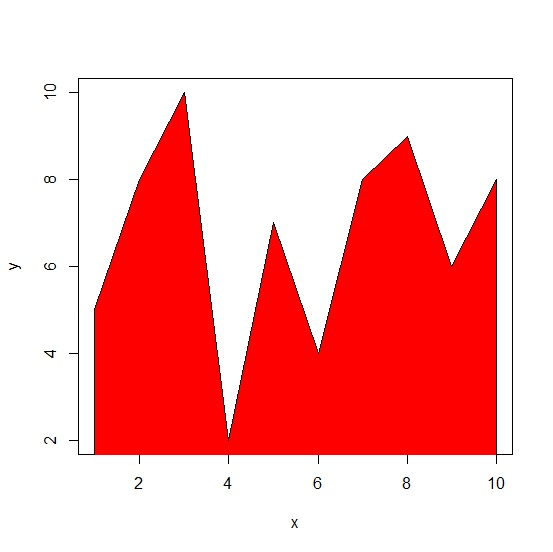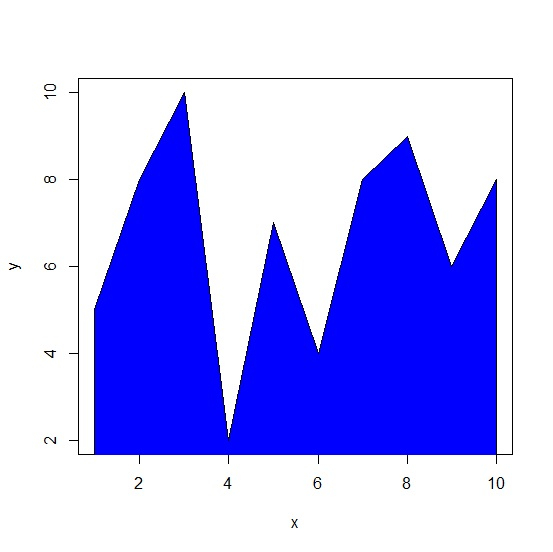# How to create a frequency polygon in R?

Frequency polygons are the graphs of the values to understand the shape of the distribution of the values. They are useful in comparing different data sets and visualising cumulative frequency distribution of the data sets. In base R, we can use polygon function to create the frequency polygon but first we should create a line plot for the two variables under consideration.

## Example

Consider the below vectors x and y −

set.seed(999)
x<-1:10
y<-sample(2:10,10,replace=TRUE)

Creating the frequency polygon with red color −

plot(x,y,type="l") polygon(c(1,x,10),c(0,y,0),col="red")

## OutputCreating the frequency polygon with blue color −

polygon(c(1,x,10),c(0,y,0),col="blue")

## OutputUpdated on: 08-Oct-2020

1K+ Views